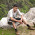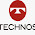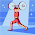## August 09, 2016

### IR Drop Analysis

Just yesterday, I got a question from one of our readers Lakshman Yandapalli. I thought it would be nice to write a blog post for you all!

Let's start with some background as to what indeed is the IR drop analysis.

When we talk about standard cells, we usually talk about the logical pins, let's say, A and B for the inputs and Z for outputs. What we do miss stating explicitly are the power/ground pins: the VDD and the VSS. These connections are usually implicit from the context (unless of course if you have a Muti-voltage design! Let's save this story for some other post).

IR drop is the voltage drop in the metal wires consituting the power grid before it reaches the VDD pins of the standard cells. Why do we bother about the voltage? Because the speed of the standard cell (the propagation delay) would be directly proportional to the VDD value. Higher VDD would mean faster cell, or lower propagation delay.

Now imagine that your SoC has a nominal voltage of 1V, and you closed your setup timing assuming the ideal 1V libraries. However, the IR drop of 40mV came into picture after you built the power grid, and the voltage is no longer 1V, let's say it is 0.96V. Now, with V = 0.96V, the delays of standard cells would be higher and you might see an increase in your setup-time violations!

Let's look into the factors that could cause this IR drop and how can we mitigate those factors, and what should our sign-off corners be to make sure no failures post-silicon!

While considering IR drop, you'd be concerned with two factors:

1. Static IR Drop: Dependent on the RC of the power grid connecting the power supply to the respective standard cells.

It is ALWAYS desirable to create the POWER GRID in higher metal layers. Higher metal layers would mean more wide wires, and hence would mean lower resistance. Lower resistance would mean that the IR drop would be lower, and hence lesser impact on setup-timing.

Capacitance of metal wires would be the combination of ground and the coupling capacitance. If for some reason, you feel that the capacitance is too large, and it is indeed the reason for IR drop, it could either be because
• Long wire length: Resulting in higher wire cap.
• High fan-out of the net: Resulting in higher load-cap, or perhaps
• High routing congestion in a particular area resulting in high coupling capacitance with the neighboring nets.

Now, how to mitigate the problem? You can try splitting the net so that the fan-out gets distributed (pretty much similar to building a clock tree), you can split the long wire by placing appropriate power bumps. Or you can also analyze the congestion and space the wires apart to reduce coupling capacitance!
Update:
Simple equation representing the static IR drop would be the following:
Vstatic_drop = Iavg x Rwire
2. Dynamic IR Drop: Dependent on the switching activity of the standard cells themselves.
Switching activity of standard cells also contributes significantly to the IR drop, also known as the Dynamic IR drop. Higher would be the switching activity, in a given region, there'll be an increased demand for current from the power supply. More is the current, more would be the IR drop (which is essentially Current times the wore resistance!).
If you ever come across such a use case, you might want to space the standard cells apart so that the burden on a given bump to feed many standard cells which have high switching activity would be mitigated.

Dynamic IR Drop is also sometimes referred to by the term of Voltage "Droop".

Update: Dynamic IR drop is contingent upon the current drawn by the standard cells, and that brings in a time-dependent variation of current into picture. Dynamic IR drop is represented by the equation:

Vdynamic_drop = L (di/dt)

Now that we have a fair understanding of IR Drop analysis, let's talk about the PVT/RC corners where one should analysis IR drop in their design.
Let's start with the RC corner.

1. RC Corner: The RC corner where the physical design engineers should analyze for IR drop would be the case when the RC product is worst. And that would indeed be the (RC)max corner, also referred to as the RCWorst corner.

2. PVT Conditions: PVT conditions would typically impact the standard cells. For IR drop analysis we would be concerned about the case where we expect the highest switching activity for standard cells. That would be the FF corner, High voltage, and high temperature.
High temperature might seem an anomaly, but higher temperature would mean higher wire resistance as well, and hence higher RC!

Last comment about IR drop analysis. It also makes sense to run IR drop analysis for the worst case setup timing check because IR drop would most probably impact only setup timing. So, designers may want to run the IR drop analysis for the RCWorst, High Temperature, SS slow and low voltage. But typically it is not done because the low voltage corner is usually already guard-banded to account for the IR drop. So, running IR drop analysis on the low voltage corner would be overly pessimistic!

#### 24 comments:

1.When voltage reduces to 0.96, is it a typo there that the delays would be lower, delays should be more, right?

1.Correct! Updated!
Thank you so much for pointing the typo! :)

-Naman

2.This comment has been removed by the author.

3.How standard cell delay depends on Voltage?

2.Nice analysis gupta. Will be more attractive with some equations related to IR.

1.Thanks for the feedback! I have a few equations in mind. Will try to update the post shortly. :)

2.Added a few equations, and will take a note of it to look for opportunities to add wherever possible.

3.4.Nice write-up. what you mean by " low voltage corner is usually already guard-banded to account for the IR drop" ?

1.Hi Vivek,
Let's say that 1V is my typical (nominal) voltage, and the best corner voltage is 1.1V, and worst corner voltage is 0.9V..
So, the 0.9V would take into account the 100mV of worst case static and dynamic IR drop itself.

5.Hi, I have this question for a long time. While analyzing the wire sizing, as you mentioned above increase in the width decreases resistance thus IR drop decreases, and increasing the width increases capacitance thus IR drop increases. So, which effect do we consider and why? Thank you.

1.Hi Naman,

Plz answer this !

2.Hello folks,
If you make the wires wider, the resistance would decrease. And yes, your observation is absolutely correct that the capacitance (though one must ask here which capacitance?) would increase. Increasing the width of the wire won't affect the coupling capacitance between the wires which usually is the main contributor towards the dynamic IR drop phenomenon.
Increasing the width of the wires would perhaps increase the fringe capacitance, which is usually too small in comparison to the coupling capacitance. Moreover, another point to be noted is how the metal layers are actually laid. Any two metal layers are orthogonal to each other, hence there's a small fringe capacitance between adjacent layers, and non-adjacent layers like m2 and m4; m3 and m5 and so on would be quite far, thereby not impacting much.

Thanks,
Naman

6.Hi, Please answer above question?
while analyzing the wire sizing, as you mentioned above increase in the width decreases resistance thus IR drop decreases, and increasing the width increases capacitance thus IR drop increases. So, which effect do we consider and why?

7.Hi Gupta,
In PVT conditions you said IR drop analysis will be on FF and high voltage and temp.
But in last comment you said that IR analysis will be on SS and low voltage, can you please clarify this.

1.IR drop analysis is done on FF and high voltage, high temperature corners because they create high switching activity which impacts dynamic IR.
SS process corner, low voltage analysis is more for timing to understand the IR impact on setup timing

8.Good information. it increase my knowledge about IR. thanks for sharing

9.why should we run Ir drop on high voltage rather then low voltage ; i think when it will pass on low voltage then it should pass on high voltage automatically?? correct me if i am wrong

10.Hi Gupta,
I have one doubt. If we decrease resistance (by upsizing wire width) then automatically current will increase (I=Vdd/R) and hence IR product should remain same . Isnt it? please correct me...

1.Can anyone explain this doubt ?
I also have the same thought in my mind .

Hello Naman, request to explain this in detail.

2.See, you're confusing the cause and effect here. Current is not the effect, it's the cause! If you decrease resistance, current would still be the same because current originated from the VDD of the device. However, by decreasing the resistance, the product IR decreases and that helps with lowering the IR drop. Or you can say the wire can now carry higher current to produce the same voltage drop.

-Naman

11.Hi, What do you mean by creating a power grid in a higher metal layer? Dont you have to bring power all the way down to the cells? If we have to bring power down to the cells, shouldn't we use all the metal layers to bring the power to the cells. Lets say the higherst metal layer is m10 for example. The cells are all placed below the metal layers. SO not the power should be brought all the way from m10 to m1 and eventually to the cells.

Can you please explain?

1.Hi, In every SOC designs we have power rings/strappings right? which means that the Power Strapping (Power Ring and Power Strap) will be much better to use higher metals let's say M10 because we can maximize the metal width based on the electrical parameters of technology. Also, in standard cells we will be creating a horizontal/vertical power rails which can be M1 or M2 (Depends on the direction of metal). Remember that we have vias in order to connect the M10 down to M1/M2. Power straps/rings or basically GRID depends on the IR Drop analysis during the floorplanning.

12.Hello Naman Gupta. I have been following your blog from long time now.I find the information you share is remarkable.
I am searching for information on RTL scripting.What are the key things to be shed light upon carrying out RTL synthesis.What are the different RTL designs can we have? And what are the various configurations used , differ for different designs?